# mysql50解法与思考过程

--建表
--学生表
CREATE TABLE Student(
s_id VARCHAR(20),
s_name VARCHAR(20) NOT NULL DEFAULT '',
s_birth VARCHAR(20) NOT NULL DEFAULT '',
s_sex VARCHAR(10) NOT NULL DEFAULT '',
PRIMARY KEY(s_id)
);

--课程表
CREATE TABLE Course(
c_id VARCHAR(20),
c_name VARCHAR(20) NOT NULL DEFAULT '',
t_id VARCHAR(20) NOT NULL,
PRIMARY KEY(c_id)
);

--教师表
CREATE TABLE Teacher(
t_id VARCHAR(20),
t_name VARCHAR(20) NOT NULL DEFAULT '',
PRIMARY KEY(t_id)
);

--成绩表
CREATE TABLE Score(
s_id VARCHAR(20),
c_id VARCHAR(20),
s_score INT(3),
PRIMARY KEY(s_id,c_id)
);

#学生表
insert into student values('01' , '赵雷' , '1990-01-01' , '男');
insert into student values('02' , '钱电' , '1990-12-21' , '男');
insert into student values('03' , '孙风' , '1990-05-20' , '男');
insert into student values('04' , '李云' , '1990-08-06' , '男');
insert into student values('05' , '周梅' , '1991-12-01' , '女');
insert into student values('06' , '吴兰' , '1992-03-01' , '女');
insert into student values('07' , '郑竹' , '1989-07-01' , '女');
insert into student values('08' , '王菊' , '1990-01-20' , '女');

#课程表
insert into course values('01' , '语文');
insert into course values('02' , '数学');
insert into course values('03' , '英语');

#教师表
insert into teacher values('01' , '张三','01');
insert into teacher values('02' , '李四','02');
insert into teacher values('03' , '王五','03');

#成绩表
insert into grade values(1,80,'01' , '01');
insert into grade values(2,90,'01' , '02');
insert into grade values(3,99,'01' , '03');
insert into grade values(4,70,'02' , '01');
insert into grade values(5,60,'02' , '02');
insert into grade values(6,80,'02' , '03');
insert into grade values(7,80,'03' , '01');
insert into grade values(8,80,'03' , '02');
insert into grade values(9,80,'03' , '03');
insert into grade values(10,50,'04' , '01');
insert into grade values(11,30,'04' , '02');
insert into grade values(12,20,'04' , '03');
insert into grade values(13,76,'05' , '01');
insert into grade values(14,87,'05' , '02');
insert into grade values(15,31,'06' , '01');
insert into grade values(16,34,'06' , '03');
insert into grade values(17,89,'07' , '02');
insert into grade values(18,98,'07' , '03');

#1、查询"语文"课程比"数学"课程成绩高的学生的信息及课程分数

select t1.s_score as '语文',t2.s_score as '数学',t1.s_id,t3.* from
(select s_score,s_id from score where c_id ='01') t1,
(select s_score,s_id  from score where c_id ='02') t2, student t3 where t1.s_id=t2.s_id and t1.s_id=t3.s_id and t1.s_score <t2.s_score

SELECT
st.*,
sc.s_score AS '语文',
sc2.s_score '数学'
FROM
student st
LEFT JOIN score sc ON sc.s_id = st.s_id
AND sc.c_id = '01'
LEFT JOIN score sc2 ON sc2.s_id = st.s_id
AND sc2.c_id = '02'
WHERE
sc.s_score > sc2.s_score
#2、查询"语文"课程比"数学"课程成绩低的学生的信息及课程分数

select t1.s_score as '语文',t2.s_score as '数学',t1.s_id,t3.s_name from
(select s_score,s_id from score where c_id ='01') t1,
(select s_score,s_id  from score where c_id ='02') t2, student t3 where t1.s_id=t2.s_id and t1.s_id=t3.s_id and t1.s_score <t2.s_score

#3、查询平均成绩大于等于60分的同学的学生编号和学生姓名和平均成绩

mysql group by没有字段限制，即group by的字段与映射列无约束，注意having和group by 和where的位置关系

select avg(s_score),t1.s_id,t3.s_name from  score t1,student t3 where t1.s_id=t3.s_id  group by t1.s_id having avg(s_score)>60;

SELECT
st.s_id,
st.s_name,
ROUND( AVG( sc.s_score ), 2 ) "平均成绩"
FROM
student st
LEFT JOIN score sc ON sc.s_id = st.s_id
GROUP BY
st.s_id
HAVING
AVG( sc.s_score )>= 60
#4查询平均成绩小于60分的同学的学生编号和学生姓名和平均成绩(包括有成绩的和无成绩的)
select avg(s_score),t1.s_id,t3.s_name from  score t1,student t3 where t1.s_id=t3.s_id  group by t1.s_id having avg(s_score)<60;
SELECT
st.s_id,
st.s_name,(
CASE

WHEN
ROUND( AVG( sc.s_score ), 2 ) IS NULL
THEN
0
ELSE
ROUND( AVG( sc.s_score ), 2 )
END
) "平均成绩"
FROM
student st
LEFT JOIN score sc ON sc.s_id = st.s_id
GROUP BY
st.s_id
HAVING
AVG( sc.s_score )< 60
OR AVG( sc.s_score ) IS NULL

#5、查询所有同学的学生编号、学生姓名、选课总数、所有课程的总成绩

select sum(t1.s_score),t1.s_id,count(t1.c_id),t2.* from  score t1 ,student t2 where t1.s_id=t2.s_id group by t1.s_id;
SELECT
st.s_id,
st.s_name,
count( sc.c_id ) "选课总数",
sum( CASE WHEN sc.s_score IS NULL THEN 0 ELSE sc.s_score END ) "总成绩"
FROM
student st
LEFT JOIN score sc ON st.s_id = sc.s_id
GROUP BY
st.s_id
#6、查询"墨"姓老师的数量

SELECT
t.t_name,
count( t.t_id )
FROM
teacher t
GROUP BY
t.t_id
HAVING
t.t_name LIKE "墨%";
# 7、查询学过"墨白"老师授课的同学的信息
select *from student where s_id in
(select s_id from score where c_id =
(select c_id from course where t_id =
(select t_id from  teacher where t_name='墨白')))

SELECT
st.*
FROM
student st
LEFT JOIN score sc ON sc.s_id = st.s_id
LEFT JOIN course c ON c.c_id = sc.c_id
LEFT JOIN teacher t ON t.t_id = c.t_id
WHERE
t.t_name = "墨白"
# 8、查询没学过"柠檬"老师授课的同学的信息
select *from student where s_id not in
(select s_id from score where c_id =
(select c_id from course where t_id =
(select t_id from  teacher where t_name='柠檬')))

SELECT
st.*
FROM
student st
WHERE
st.s_id NOT IN (
SELECT
sc.s_id
FROM
score sc
WHERE
sc.c_id IN ( SELECT c.c_id FROM course c LEFT JOIN teacher t ON t.t_id = c.t_id WHERE t.t_name = "柠檬" )
)
#9、查询学过编号为"01"并且也学过编号为"02"的课程的同学的信息
select s_id from  score  where s_id in
(select s_id from  score where c_id in ('01')) and c_id in ('02');

select * from  student where  s_id in (
select s_id from  score  where s_id in
(select s_id from  score where c_id in ('02')) and c_id in ('01'));

update student set s_name = '李嘉欣' , s_birth = '1979-09-12' , s_sex = '女',moble ='11111111111' where s_id = 18;
select * from  course;
select * from score;
select * from student;
select * from student;
insert into student(s_name,s_birth,s_sex) values('李宗盛','1991-08-16','123456');

delete from student where s_id='8' and s_name ='李嘉欣';
select sum(t1.s_score),t1.s_id,count(t1.c_id),t2.* from  score t1 ,student t2 where t1.s_id=t2.s_id group by t1.s_id

10、查询学过编号为"01"但是没有学过编号为"02"的课程的同学的信息

select s_id from  score where c_id in ('01') and s_id in(
select s_id from  score where s_id not in(
select s_id from  score where c_id  in ('02')))

11、查询没有学全所有课程的同学的信息

select * from  student where s_id not in(
select s_id from  score  group by s_id having count(c_id)=3)

12、查询至少有一门课与学号为"01"的同学所学相同的同学的信息

select * from student where s_id in(
select s_id from  score where c_id in(
select c_id from  score where s_id ='01'))

13、查询和"01"号的同学学习的课程完全相同的其他同学的信息

sql合并字段值_萧木易的博客-CSDN博客

SELECT GROUP_CONCAT(c_id),s_id FROM  score GROUP BY s_id

SELECT GROUP_CONCAT(c_id),s_id FROM  score GROUP BY s_id HAVING s_id='01'

SELECT s_id  FROM
(SELECT GROUP_CONCAT(c_id) all_c_id,s_id FROM  score GROUP BY s_id) t1 WHERE all_c_id=(SELECT GROUP_CONCAT(c_id) all_c_id FROM  score GROUP BY s_id HAVING s_id='01')

SELECT * FROM student WHERE s_id IN
(SELECT s_id  FROM
(SELECT GROUP_CONCAT(c_id) all_c_id,s_id FROM  score GROUP BY s_id) t1 WHERE all_c_id=(SELECT GROUP_CONCAT(c_id) all_c_id FROM  score GROUP BY s_id HAVING s_id='01'))
AND s_id!='01'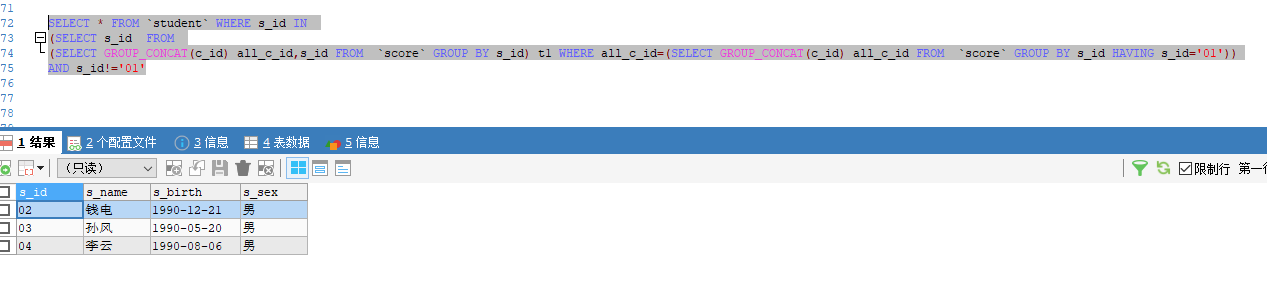14、查询各科成绩最高分、最低分和平均分： 以如下形式显示：

SELECT MAX(s_score),AVG(s_score),MIN(s_score),c_id FROM score GROUP BY c_id

SELECT
CASE WHEN s_score>=90  THEN  '优秀'
WHEN s_score>=80  THEN  '优良'
WHEN s_score>=70  THEN  '中等'
WHEN s_score>=60  THEN  '及格'
ELSE '不及格'
END '成绩',
c_id,s_id
FROM score GROUP BY c_id,s_id

SELECT  SUM(CASE WHEN s_score>=90  THEN  1     ELSE 0 END ) '优秀',
SUM(CASE WHEN s_score>=80 AND  s_score<90 THEN  1     ELSE 0 END ) '优良',
SUM(CASE WHEN s_score>=70 AND  s_score<80 THEN  1     ELSE 0 END ) '中等',
SUM(CASE WHEN s_score>=70 AND  s_score<80 THEN  1     ELSE 0 END ) '及格',
SUM(CASE WHEN s_score<60 THEN  1     ELSE 0 END ) '不及格',
c_id FROM score GROUP BY c_id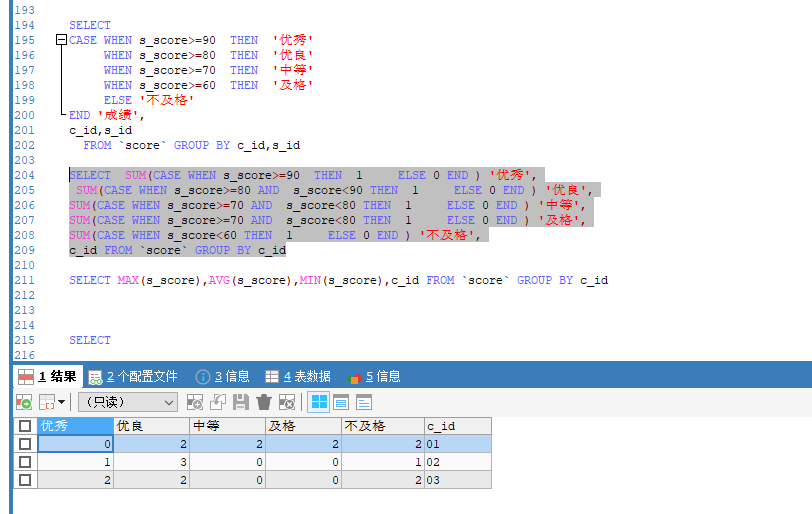SELECT  (SUM(CASE WHEN s_score>=90  THEN  1     ELSE 0 END ))/COUNT(c_id) '优秀',
(SUM(CASE WHEN s_score>=80 AND  s_score<90 THEN  1     ELSE 0 END ))/COUNT(c_id) '优良',
(SUM(CASE WHEN s_score>=70 AND  s_score<80 THEN  1     ELSE 0 END ))/COUNT(c_id) '中等',
(SUM(CASE WHEN s_score>=60 AND  s_score<70 THEN  1     ELSE 0 END ))/COUNT(c_id) '及格',
(SUM(CASE WHEN s_score<60 THEN  1     ELSE 0 END ))/COUNT(c_id) '不及格',
c_id,COUNT(c_id) FROM score GROUP BY c_id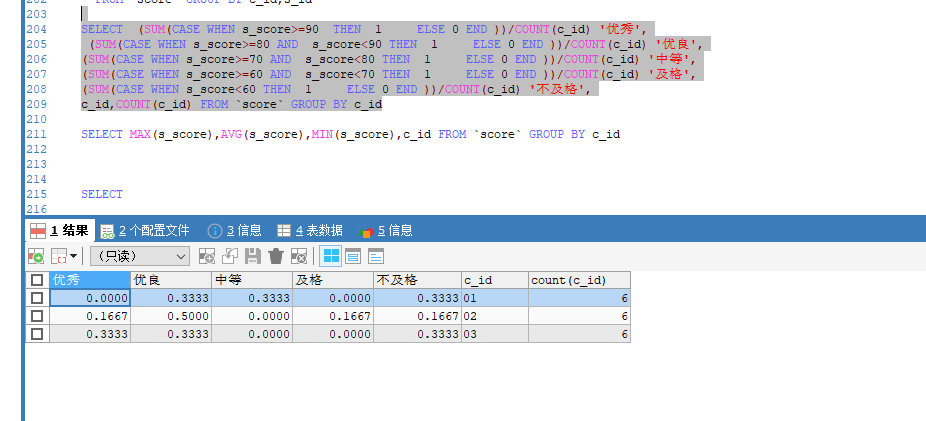和最高分、最低分和平均分sql合并

SELECT   MAX(s_score),AVG(s_score),MIN(s_score),
(SUM(CASE WHEN s_score>=90  THEN  1     ELSE 0 END ))/COUNT(c_id) '优秀',
(SUM(CASE WHEN s_score>=80 AND  s_score<90 THEN  1     ELSE 0 END ))/COUNT(c_id) '优良',
(SUM(CASE WHEN s_score>=70 AND  s_score<80 THEN  1     ELSE 0 END ))/COUNT(c_id) '中等',
(SUM(CASE WHEN s_score>=60 AND  s_score<70 THEN  1     ELSE 0 END ))/COUNT(c_id) '及格',
(SUM(CASE WHEN s_score<60 THEN  1     ELSE 0 END ))/COUNT(c_id) '不及格',
c_id,COUNT(c_id) FROM score GROUP BY c_id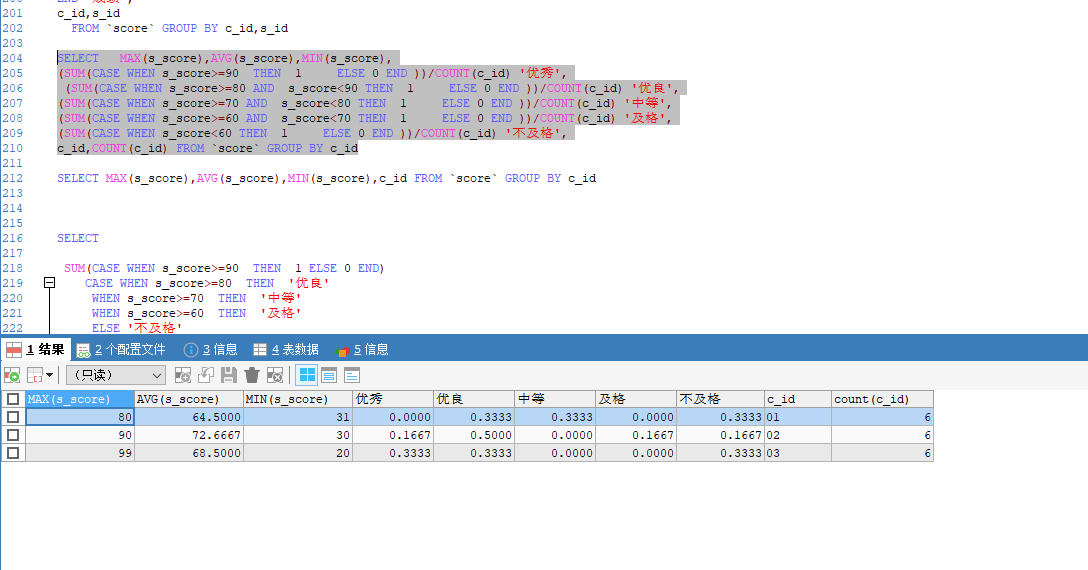15、按各科成绩进行排序，并显示排名， Score 重复时保留名次空缺

SELECT
CASE WHEN s_score>=90  THEN  '优秀'
WHEN s_score>=80  THEN  '优良'
WHEN s_score>=70  THEN  '中等'
WHEN s_score>=60  THEN  '及格'
ELSE '不及格'
END '成绩',s_score,
c_id
FROM score GROUP BY c_id,s_score ORDER BY c_id,s_score DESC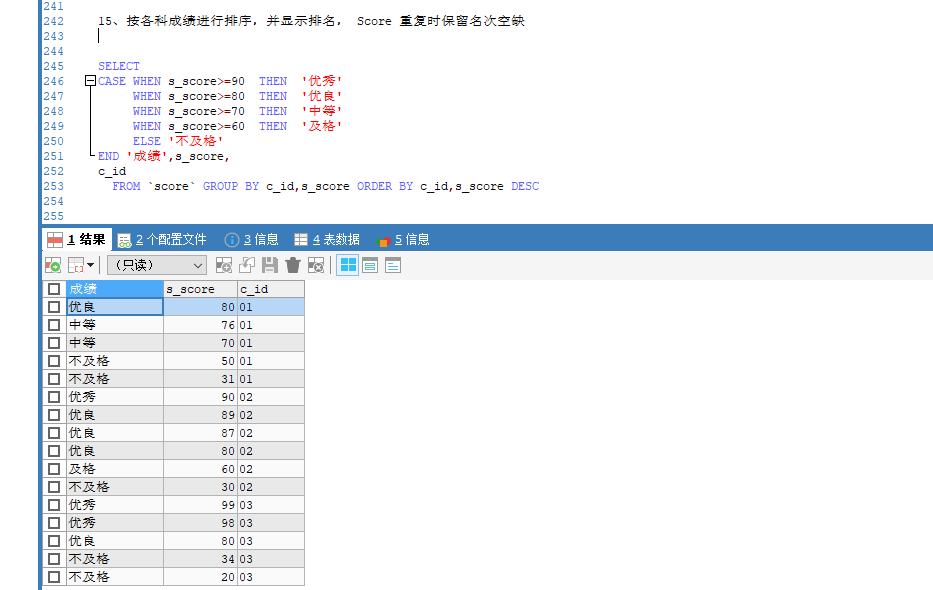SELECT
@i:=@i +1 AS i ,
score.c_id,
score.s_score,
score.s_id
FROM (SELECT @i:=0)s,(SELECT * FROM score GROUP BY c_id,s_id ORDER BY c_id,s_score DESC) score;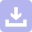下载
mysql经典50题
04-01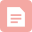博客
MySQL经典练习50题
10-291万+博客
MySQL 50题
04-082343博客
MySQL 50条必练查询语句
07-067万+博客

04-291701博客
mysql基本50题_MySQL经典50题
01-19194博客
50道SQL练习题及答案详细分析
03-022759博客
MySQL50题练习

01-07626博客
mysql50._关于mysql中库名为#mysql50#的问题
01-19291博客
MySQL数据库连接时，出现名为#mysql50#的数据库
01-06178博客
#mysql50#_关于mysql中库名为#mysql50#的问题
03-0589博客
mysql经典50题（练习自用版）
03-301124博客
MYSQL中出现名为#mysql50#的数据库，解决办法
04-172430博客
MySQL 50道练习题及答案
03-306135博客
10-0380博客

06-03303博客
MySql 50句及解析
09-181419博客
sql语句练习50题(Mysql版)

12-1935万+博客
MySQL面试50题和答案
11-301万+

### “相关推荐”对你有帮助么？

•非常没帮助
•没帮助
•一般
•有帮助
•非常有帮助¥2 ¥4 ¥6 ¥10 ¥20余额支付 (余额：-- )扫码支付获取中扫码支付点击重新获取扫码支付1.余额是钱包充值的虚拟货币，按照1:1的比例进行支付金额的抵扣。
2.余额无法直接购买下载，可以购买VIP、C币套餐、付费专栏及课程。余额充值